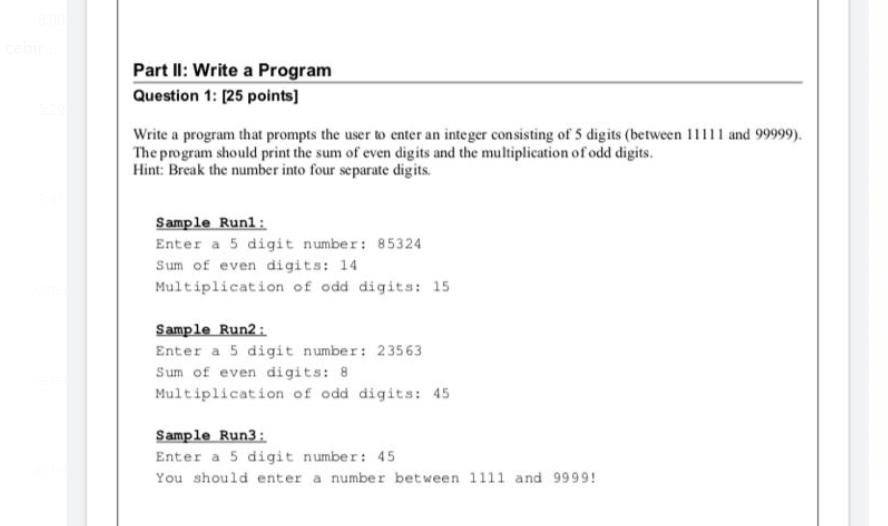## Part II: Write a Program Question 1: [25 points] Write a program that prompts the user to enter a...Part II: Write a Program Question 1: [25 points]
Write a program that prompts the user to enter an integer consisting of 5 digits (between 11111 and 99999). The program should print the sum of even digits and the multiplication of odd digits.
Hint: Break the number into four separate digits.
Sample Run1:
Enter a 5 digit number: 85324
Sum of even digits: 14
Multiplication of odd digits: 15
Sample Run2:
Enter a 5 digit number: 23563
Sum of even digits: 8
Multiplication of odd digits: 45
Sample Run3:
Enter a 5 digit number: 45
You should enter a number between 1111 and 9999!

Introduction to programming

Solved
Programming in C,C++ 1 Answer Aneesh Matlani